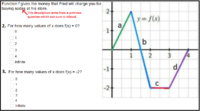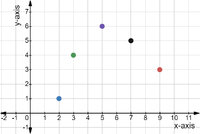# Really confused how to answer this question on how many x does f(x)=0 on a graph.

#### Rhodium

##### New member
The graph structure came from a previous question so I added the detail about it being about sodas. How ever we don't really know if the story carries over from the previous question.. the previous was just asking if it was increasing, decreasing or constant for each segment. The value go negative which means giving money?? So that being said...For the first question, our thinking is that the graph crosses x axis 3 times.. so we think 3 would be the right answer. However if you have to only select integers, then it would be 2. What is also confusing is that you can select multiple answers which I think there would only be one.

For the second question.. it is more confusing to us. I say the value is 2, but my son thinks 3.

Thank you for your time and insight on this problem!

#### HallsofIvy

##### Elite Member
Basically you are asking about how to read a graph?

The first question is "How many times is f(x)= 0?" The graph specifically says that "y= f(x)" so that is the same as y= 0 and thar is, as you say, the x- axis. Yes, the graph crosses the x-axis three times so y= f(x)= 0 three times.

You say "if you have to only select integers, then it would be 2".
Why would you even think about integers? The graph says nothing about integers!
f(x)= 0 for x= 0, 3/2, and 4.

The next question asks "For how many values of x does f(x)= -2." You say "2" (I presume you are referring to x= 2 and x= 3) while your son says "3" (I presume because there is a third vertical line at x= 2.5.)

Purely from the graph, neither is correct! EVERY value of x between 2 and 3 gives f(x)= -2. The correct answer is the last one- "infinite".

But I understand your confusion! I am answering purely from the point of view of reading the graph. The "story" that accompanies the problem is simply ridiculus! Certainly Fred will NOT charge you a negative amount of money (i.e. pay you) if you buy two or three sodas and it doesn't make sense to buy 3/2 soda. If we rely on that the problem is nonsense- so don't- just read the graph!

•topsquark and tikA

#### Rhodium

##### New member
Thank you for taking your time to help answer this.

For the first on why I thought about integers was due to x axis being sodas, hence the points would only be at every changing value. Hence the 2.5 is just the passing through of the y=0 point, but not an official measurement point. I felt 3 was correct until I looked at the second question.. and possibly overread it.

You mention the 2nd question should be infinite.. this doesn't make sense to me. Infinite implies that it wouldn't be any other value other than -2. Hence it would be one straight line. Now if the question only focused on segment c then I would agree infinite would make some sense.

#### Jomo

##### Elite Member
There are an infinite number of number between 2 and 3! For example consider the following DIFFERENT numbers between 2 and 3: 2 1/2, 2 1/3, 2 1/4, 2 1/5, 2 1/6, 2 1/7, 2 1/8, 2 1/9, 2 1/10, 2 1/11, ...
That is an infinite number of numbers all between 2 and 3.

Just out of curiosity, how many numbers did you think there are between 2 and 3??

#### Rhodium

##### New member
I get that there are infinite value between 2 and 3, but the 3rd question didn't say look at specific segment.. so that's where it confused me.
Now looking at it in a different way... I think I see what you both of you are talking about. I think I was having a hard time interpreting the question.

#### Zermelo

##### Junior Member
Thank you for taking your time to help answer this.

For the first on why I thought about integers was due to x axis being sodas, hence the points would only be at every changing value. Hence the 2.5 is just the passing through of the y=0 point, but not an official measurement point. I felt 3 was correct until I looked at the second question.. and possibly overread it.

You mention the 2nd question should be infinite.. this doesn't make sense to me. Infinite implies that it wouldn't be any other value other than -2. Hence it would be one straight line. Now if the question only focused on segment c then I would agree infinite would make some sense.
Yes that makes sense, but look at the graph! The graph is continious, it it were only integers like you say, then the graph wouldn’t be straight lines but dots!That’s what the graph looks like if the function domain is the natural numbers. In your graph, every number between 2 and 3 satisfies the condition. 2.01, 2.001, 2.0001,... there are even infinite numbers between 2 and 2.1•Jomo, JeffM and Subhotosh Khan

#### lookagain

##### Elite Member
Yes, the graph crosses the x-axis three times so y= f(x)= 0 three times.

It looks like the graph "crosses" the x-axis once between x = 1 and x = 2 and "touches" at x = 0 and x = 4 for
a total of three x-intercepts.

#### Rhodium

##### New member
BTW I forgot to come back and thank you all again for the help. (I didn't mean to come here ask and run)

•Jomo

#### Jomo

##### Elite Member
The real problem is that this graph does not reflect the story which it came from. The number of sodas which you can buy is not continuous--you can not buy pi cans of soda. Likewise you can't buy 2 1/2 cans of soda. So the graph does not match the story. Also, as already pointed out, you will not be paid to buy 2 or 3 cans of soda! Personally, I would stay in the store all day long buying 2 cans of soda at a time (why not 3 cans?) until they were sold out. Actually, I would then sell the owner back the cans of soda for a reasonable fee--and buy two at a time again--and again!

•HallsofIvy